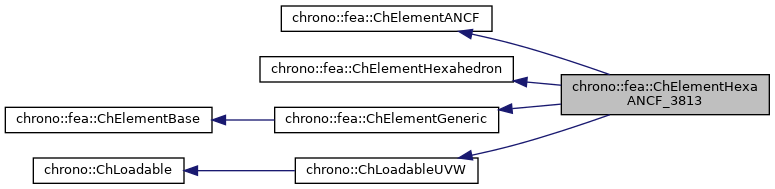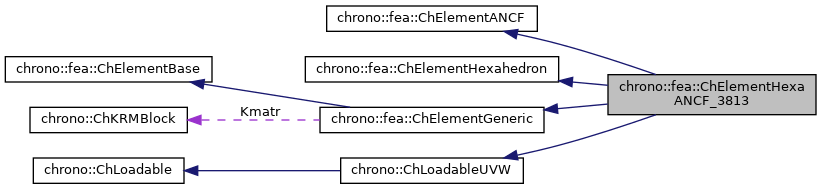chrono::fea::ChElementHexaANCF_3813 Class Reference

## Description

Hexahedronal solid element with 8 nodes (with EAS).

While technically not an ANCF element, the name is justified because the implementation can use the same ANCF machinery.

#include <ChElementHexaANCF_3813.h>

Inheritance diagram for chrono::fea::ChElementHexaANCF_3813:[legend]
Collaboration diagram for chrono::fea::ChElementHexaANCF_3813:[legend]

## Public Types

using ShapeVector = ChMatrixNM< double, 1, 8 >

## Public Member Functions

virtual int GetNnodes () override
Get number of nodes of this element.

virtual int GetNdofs () override
Get the number of coordinates in the field used by the referenced nodes.

virtual int GetNdofs_active () override
Get the number of active coordinates in the field used by the referenced nodes.

virtual int GetNodeNdofs (int n) override
Get the number of coordinates from the n-th node used by this element.

virtual int GetNodeNdofs_active (int n) override
Get the number of active coordinates from the n-th node used by this element.

virtual std::shared_ptr< ChNodeFEAbaseGetNodeN (int n) override
Access the n-th node of this element.

virtual std::shared_ptr< ChNodeFEAxyzGetHexahedronNode (int n) override
Return the specified hexahedron node (0 <= n <= 7).

void SetNodes (std::shared_ptr< ChNodeFEAxyz > nodeA, std::shared_ptr< ChNodeFEAxyz > nodeB, std::shared_ptr< ChNodeFEAxyz > nodeC, std::shared_ptr< ChNodeFEAxyz > nodeD, std::shared_ptr< ChNodeFEAxyz > nodeE, std::shared_ptr< ChNodeFEAxyz > nodeF, std::shared_ptr< ChNodeFEAxyz > nodeG, std::shared_ptr< ChNodeFEAxyz > nodeH)
Specify the nodes of this element.

void SetElemNum (int kb)
Set the element number.

void SetStockAlpha (double a1, double a2, double a3, double a4, double a5, double a6, double a7, double a8, double a9)
Set EAS internal parameters (stored values).

void SetInertFlexVec (const ChVector< double > &a)
Set some element parameters (dimensions).

int GetElemNum () const

const ChMatrixNM< double, 8, 3 > & GetInitialPos () const
Get initial position of the element in matrix form.

std::shared_ptr< ChNodeFEAxyzGetNodeA () const

std::shared_ptr< ChNodeFEAxyzGetNodeB () const

std::shared_ptr< ChNodeFEAxyzGetNodeC () const

std::shared_ptr< ChNodeFEAxyzGetNodeD () const

std::shared_ptr< ChNodeFEAxyzGetNodeE () const

std::shared_ptr< ChNodeFEAxyzGetNodeF () const

std::shared_ptr< ChNodeFEAxyzGetNodeG () const

std::shared_ptr< ChNodeFEAxyzGetNodeH () const

double GetLengthX () const

double GetLengthY () const

double GetLengthZ () const

void SetMaterial (std::shared_ptr< ChContinuumElastic > my_material)

std::shared_ptr< ChContinuumElasticGetMaterial () const

void SetMooneyRivlin (bool val)
Set whether material is Mooney-Rivlin (Otherwise linear elastic isotropic)

void SetMRCoefficients (double C1, double C2)
Set Mooney-Rivlin coefficients.

void ShapeFunctions (ShapeVector &N, double x, double y, double z)
Fills the N shape function matrix as N = [s1*eye(3) s2*eye(3) s3*eye(3) s4*eye(3)...]; ,.

void ShapeFunctionsDerivativeX (ShapeVector &Nx, double x, double y, double z)

void ShapeFunctionsDerivativeY (ShapeVector &Ny, double x, double y, double z)

void ShapeFunctionsDerivativeZ (ShapeVector &Nz, double x, double y, double z)

Gets the number of DOFs affected by this element (position part)

Gets the number of DOFs affected by this element (speed part)

virtual void LoadableGetStateBlock_x (int block_offset, ChState &mD) override
Gets all the DOFs packed in a single vector (position part)

virtual void LoadableGetStateBlock_w (int block_offset, ChStateDelta &mD) override
Gets all the DOFs packed in a single vector (speed part)

virtual void LoadableStateIncrement (const unsigned int off_x, ChState &x_new, const ChState &x, const unsigned int off_v, const ChStateDelta &Dv) override
Increment all DOFs using a delta.

virtual int Get_field_ncoords () override
Number of coordinates in the interpolated field: here the {x,y,z} displacement.

virtual int GetSubBlocks () override
Get the number of DOFs sub-blocks.

virtual unsigned int GetSubBlockOffset (int nblock) override
Get the offset of the specified sub-block of DOFs in global vector.

virtual unsigned int GetSubBlockSize (int nblock) override
Get the size of the specified sub-block of DOFs in global vector.

virtual bool IsSubBlockActive (int nblock) const override
Check if the specified sub-block of DOFs is active.

virtual void LoadableGetVariables (std::vector< ChVariables * > &mvars) override
Get the pointers to the contained ChVariables, appending to the mvars vector.

virtual void ComputeNF (const double U, const double V, const double W, ChVectorDynamic<> &Qi, double &detJ, const ChVectorDynamic<> &F, ChVectorDynamic<> *state_x, ChVectorDynamic<> *state_w) override
Evaluate N'*F , where N is some type of shape function evaluated at U,V,W coordinates of the volume, each ranging in -1..+1 F is a load, N'*F is the resulting generalized load Returns also det[J] with J=[dx/du,..], that might be useful in gauss quadrature. More...

virtual double GetDensity () override
This is needed so that it can be accessed by ChLoaderVolumeGravity.Public Member Functions inherited from chrono::fea::ChElementGeneric
ChKblockGenericKstiffness ()
Access the proxy to stiffness, for sparse solver.

virtual void EleIntLoadResidual_F (ChVectorDynamic<> &R, const double c) override
Add the internal forces (pasted at global nodes offsets) into a global vector R, multiplied by a scaling factor c, as R += forces * c This default implementation is SLIGHTLY INEFFICIENT.

virtual void EleIntLoadResidual_Mv (ChVectorDynamic<> &R, const ChVectorDynamic<> &w, const double c) override
Add the product of element mass M by a vector w (pasted at global nodes offsets) into a global vector R, multiplied by a scaling factor c, as R += M * w * c This default implementation is VERY INEFFICIENT.

virtual void EleIntLoadResidual_F_gravity (ChVectorDynamic<> &R, const ChVector<> &G_acc, const double c) override
Add the contribution of gravity loads, multiplied by a scaling factor c, as: R += M * g * c This default implementation is VERY INEFFICIENT. More...

virtual void InjectKRMmatrices (ChSystemDescriptor &descriptor) override
Tell to a system descriptor that there are item(s) of type ChKblock in this object (for further passing it to a solver)

virtual void KRMmatricesLoad (double Kfactor, double Rfactor, double Mfactor) override
Add the current stiffness K and damping R and mass M matrices in encapsulated ChKblock item(s), if any. More...

virtual void VariablesFbLoadInternalForces (double factor=1.) override
Add the internal forces, expressed as nodal forces, into the encapsulated ChVariables.

virtual void VariablesFbIncrementMq () override
Add M*q (internal masses multiplied current 'qb').Public Member Functions inherited from chrono::fea::ChElementBase
virtual void ComputeNodalMass ()
Compute element's nodal masses.

virtual void EleDoIntegration ()
This is optionally implemented if there is some internal state that requires integration.Public Member Functions inherited from chrono::ChLoadableUVW
virtual bool IsTetrahedronIntegrationNeeded ()
If true, use quadrature over u,v,w in [0..1] range as tetrahedron volumetric coords (with z=1-u-v-w) otherwise use default quadrature over u,v,w in [-1..+1] as box isoparametric coords.

virtual bool IsTrianglePrismIntegrationNeeded ()
If true, use quadrature over u,v in [0..1] range as triangle natural coords (with z=1-u-v), and use linear quadrature over w in [-1..+1], otherwise use default quadrature over u,v,w in [-1..+1] as box isoparametric coords.

## Friends

class Brick_ForceAnalytical

class Brick_ForceNumericalProtected Attributes inherited from chrono::fea::ChElementANCF
int m_element_dof
actual number of degrees of freedom for the element

bool m_full_dof
true if all node variables are active (not fixed)

ChArray< int > m_mapping_dof
indices of active DOFs (set only is some are fixed)Protected Attributes inherited from chrono::fea::ChElementGeneric
ChKblockGeneric Kmatr

## ◆ ComputeNF()

 void chrono::fea::ChElementHexaANCF_3813::ComputeNF ( const double U, const double V, const double W, ChVectorDynamic<> & Qi, double & detJ, const ChVectorDynamic<> & F, ChVectorDynamic<> * state_x, ChVectorDynamic<> * state_w )
overridevirtual

Evaluate N'*F , where N is some type of shape function evaluated at U,V,W coordinates of the volume, each ranging in -1..+1 F is a load, N'*F is the resulting generalized load Returns also det[J] with J=[dx/du,..], that might be useful in gauss quadrature.

Parameters
 U parametric coordinate in volume V parametric coordinate in volume W parametric coordinate in volume Qi Return result of N'*F here, maybe with offset block_offset detJ Return det[J] here F Input F vector, size is = n.field coords. state_x if != 0, update state (pos. part) to this, then evaluate Q state_w if != 0, update state (speed part) to this, then evaluate Q